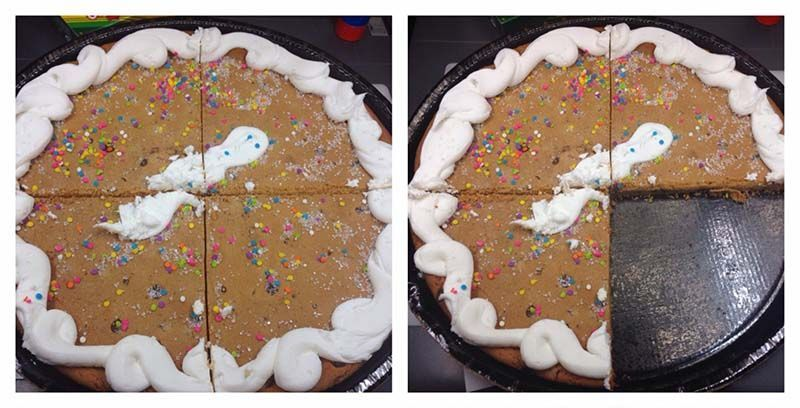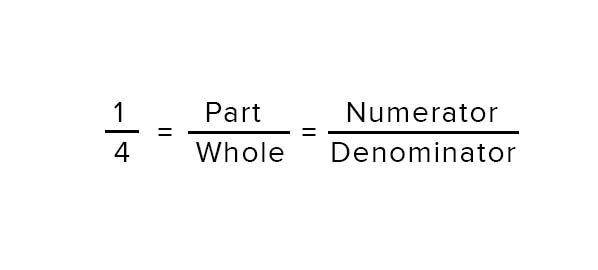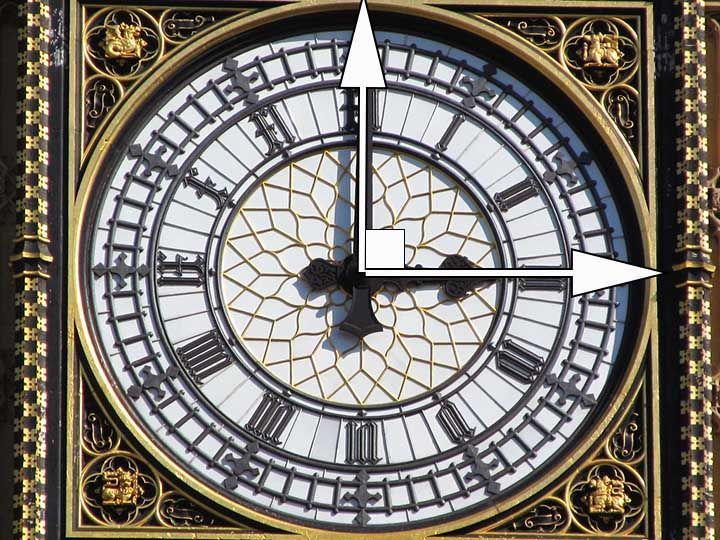# Frcations.A fraction simply tells us how many equal parts of a whole we have.

Let's watch a video to understand what fractions are.

# Fractions is. Making Parts.

Tom, Jerry and Spike are fighting because they didn't know how to divide the meat into equal parts.

Fractions help us divide things into equal parts, so that we don't fight like Tom, Jerry and Spike.

Fractions are formed when we divide a whole into equal parts.

Each equal part of the whole is called a fraction.To represent this part of the whole, we write it as 1 over 4.

Where the number above the horizontal line represents the parts and the number below the horizontal line represents the whole.

The number above the horizontal line which represents the parts is called the numerator.

And the number below the horizontal line which represents the whole is called the denominator.

# Access All Content

## More from Grade 6 Math# Types of Lines

All you need to know about lines, parallel, perpendicular and intersecting lines. Along with all the examples from the world around you.# Types of Angles

Learn about the different types of angles acute, right, obtuse and more through exciting facts from the Wonders of the World.# Celsius to Fahrenheit

Learn how to check the temperature, anytime and anywhere.